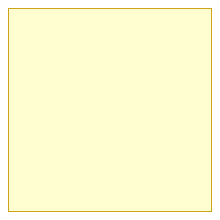Search IntMath
Close

# Square vs. Rectangle in Geometry## What is a Square?

A square is a four-sided shape with all sides equal in length. It is a special type of quadrilateral, and its angles are all right angles. A square is both a rectangle and a parallelogram. It�s a very important shape in geometry.

A square can be defined as a four-sided shape with all four sides having the same length. The angles of a square are all right angles, meaning they are all 90 degrees. Additionally, a square is a type of rectangle and a type of parallelogram. This means that if you have a square, you can also call it a rectangle and a parallelogram.

## What is a Rectangle?

A rectangle is a four-sided shape with two sets of parallel sides. The two pairs of parallel sides are of different lengths. The angles of a rectangle are all right angles, meaning they are all 90 degrees. It is not a parallelogram.

A rectangle can be defined as a four-sided shape with two sets of parallel sides. The two sets of parallel sides have different lengths. The angles of a rectangle are all right angles, meaning they are all 90 degrees. This means that a rectangle is not a parallelogram.

## Square vs. Rectangle

A square and a rectangle are both four-sided shapes. However, a square has all sides equal in length, while a rectangle has two sets of parallel sides of different lengths. Additionally, both a square and a rectangle are parallelograms, but a rectangle is not a square.

It is important to note that a square is a special type of rectangle. This means that if you have a square, it can also be called a rectangle. However, if you have a rectangle, it cannot be called a square.

## Practice Problems

• If a shape has four sides and four right angles, can it be both a square and a rectangle?
• Is a rectangle a parallelogram?
• If a shape has four sides of equal length, is it a square?
• If a shape has four sides of different lengths, is it a square?
• Can a square be called a rectangle?

• Yes, a shape that has four sides and four right angles can be both a square and a rectangle.
• Yes, a rectangle is a parallelogram.
• Yes, a shape that has four sides of equal length is a square.
• No, a shape that has four sides of different lengths is not a square.
• Yes, a square can be called a rectangle.

## Summary

In this article, we explored the differences between a square and a rectangle in geometry. We discovered that a square is a four-sided shape with all sides equal in length, while a rectangle is a four-sided shape with two sets of parallel sides of different lengths. Additionally, we learned that a square is a type of rectangle and a type of parallelogram, but a rectangle is not a square. Finally, we practiced these concepts with some practice problems and answers.

## FAQ

### Is a square a rectangle explain?

Yes, a square is a type of rectangle. A rectangle is a four-sided shape with its opposite sides parallel and of equal length. A square is a type of rectangle with all four sides of equal length.

### How do you prove that a rectangle is a square?

To prove that a rectangle is a square, you need to show that all four sides are of equal length. To do this, take measurements from two opposite sides of the rectangle. If the measurements are equal, then the rectangle is a square.

### Why is a square a rectangle for kids?

A square is a type of rectangle for kids because it helps them understand the concept of a rectangle. It is easier to understand the concept of a four-sided shape with two sets of parallel sides of equal length when they can visualize the shape as a square. It also helps them understand the concept of symmetry and the properties of a shape that make it a square.

## Problem SolverThis tool combines the power of mathematical computation engine that excels at solving mathematical formulas with the power of GPT large language models to parse and generate natural language. This creates math problem solver thats more accurate than ChatGPT, more flexible than a calculator, and faster answers than a human tutor. Learn More.